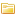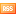# Math CategoriesAlgebraCalculusComplex VariablesDiscrete MathematicsGeometryGraph TheoryLinear & Abstract AlgebraMATLABMathematical AnalysisMathematical ChaosMathematical Logic and Set TheoryMatrixNumber TheoryOptimizationPrinciples of MathematicsStatisticsTrigonometryGet updated as we add new Math resources via our RSS feed.